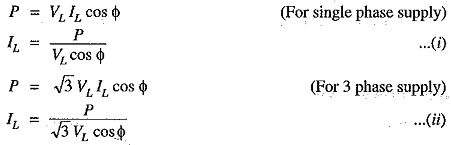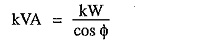## Low Power Factor:

The Low Power Factor plays an importance role in ac. circuits since power consumed depends upon this factor.It is clear from above that for fixed power and voltage, the load current is inversely proportional to the power factor. Lower the power factor, higher is the load current and vice-versa. A power factor less than unity results in the following disadvantages :

1.Large kVA rating of equipment: The electrical machinery (e.g., alternators, transformers, switchgear) is always rated in kVA.It is clear that kVA rating of the equipment is inversely proportional to power factor. The smaller the power factor, the larger is the kVA rating. Therefore, at low power factor, the kVA rating of the equipment has to be made more, making the equipment larger and expensive.

2.Greater conductor size: To transmit or distribute a fixed amount of power at constant voltage, the conductor will have to carry more current at low power factor. This necessitates large conductor size. For example, take the case of a single phase a.c. motor having an input of 10 kW on full load, the terminal voltage being 250 V. At unity p.f., the input full load current would be 10,000/250 = 40 A. At 0.8 p.f; the kVA input would be 10/0.8 = 12.5 and the current input 12,500/250 = 50 A. If the motor is worked at a low power factor of 0.8, the cross-sectional area of the supply cables and motor conductors would have to be based upon a current of 50 A instead of 40 A which would be required at unity power factor.

3.Large copper losses: The large current at low power factor causes more 12R losses in all the elements of the supply system. This results in poor efficiency.

4.Poor voltage regulation: The large current at low lagging power factor causes greater voltage drops in alternators, transformers, transmission lines and distributors. This results in the decreased voltage available at the supply end, thus impairing the performance of utilisation devices. In order to keep the receiving end voltage within permissible limits, fl extra equipment (i.e., voltage regulators) is required.

5.Reduced handling capacity of system: The lagging power factor reduces the handling capacity of all the elements of the system. It is because the reactive component of current prevents the full utilisation of installed capacity.

The above discussion leads to the conclusion that low power factor is an objectionable feature in the supply system

### Causes of Low Power Factor

Low power factor is undesirable from economic point of view. Normally, the power factor of the whole load on the supply system in lower than 0.8. The following are the causes of low power factor:

1. Most of the a.c. motors are of induction type (1Φ and 3Φ induction motors) which have low lagging power factor. These motors work at a power factor which is extremely small on light load (0.2 to 03) and rises to 0.8 or 0.9 at full load.
2. Arc lamps, electric discharge lamps and industrial heating furnaces operate at low lagging power factor.
3. The load on the power system is varying ; being high during morning and evening and low at other times. During low load period, supply voltage is increased which increases the magnetization current. This results in the decreased power factor.
Scroll to Top